• Subject:

...

• Topic:

...

A and B in the following reactions are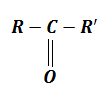$\underset{\mathrm{KCN}}{\overset{\mathrm{HCN}/}{\to }}$A$\stackrel{\mathrm{B}}{\to }$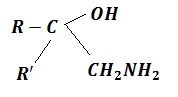(a) A=${\mathrm{RR}}^{\text{'}}{\mathrm{CH}}_{2}\mathrm{CN}$, B=NaOH

(b) A=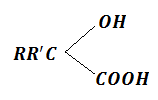, B=${\mathrm{CH}}_{3}$

(c) A=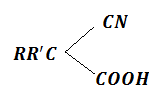, B=${\mathrm{CH}}_{3}$

(d) A=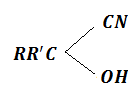, B=${\mathrm{LiAlH}}_{4}$